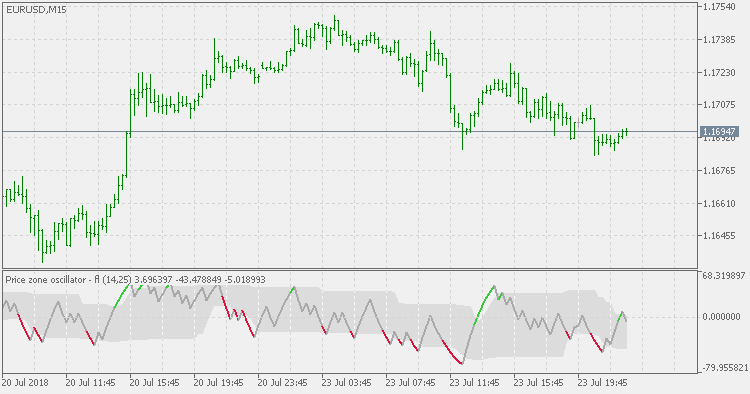Interesting script?
So post a link to it -
let others appraise it

You liked the script? Try it in the MetaTrader 5 terminal# Price Zone Oscillator - Floating Levels - indicator for MetaTrader 5

Views:
2288
Rating:
Published:
2018.07.24 12:01

Price Zone Oscillator indicator is based on the "Entering the Price Zone" article by Walid Khalil and David Steckler.

In our last article, we introduced a new oscillator that provides better guidance to buying and selling levels for securities: the Volume Zone Oscillator (VZO). This time, we introduce a similar, complementary indicator: the Price Zone Oscillator (PZO).

The formula for PZO depends on only one condition: if today's closing price is higher than yesterday's closing price, then the closing price will have a positive value (bullish); otherwise it will have a negative value (bearish).

Price Zone Oscillator = 100 x (CP/TC).

where:

CP (Closing position) = X-days EMA (± close).

and

TC (Total close) = X-days EMA (close).

Compared to the Price Zone Oscillator indicator, this version is using floating levels to find out the significant levels. To have a dynamic zero line, set both levels to 50.Price Zone Oscillator

The formula for Price Zone Oscillator (PZO) depends on only one condition: if today's closing price is higher than yesterday's closing price, then the closing price will have a positive value (bullish); otherwise it will have a negative value (bearish).Henderson's Filter

The Henderson Filters are derived by minimizing the sum of squares of the third difference of the moving average series. Henderson's criteria ensures that when these filters are applied to third degree polynomials, the resulting smoothed output will fit exactly on these parabolas. The Henderson Filters are suitable for smoothing economic time series as they allow the cycles typical of the trend to pass through unchanged. They also have the property that they will eliminate almost all the irregular variations that are of very short frequencies of six months or less.Normalized RSI

Normalized RSI tries to fix the "RSI problem": the longer the calculating period, the flatter the RSI becomes.Normalized RSI JMA Smoothed

This version of Normalized RSI is adding JMA smoothing to it in order to make that volatility lesser and to attempt to make the slope of the RSI more usable without adding significant lag.